# Test: Vector Analysis

## 10 Questions MCQ Test Topicwise Question Bank for Electronics Engineering | Test: Vector Analysis

Description
Attempt Test: Vector Analysis | 10 questions in 30 minutes | Mock test for Electrical Engineering (EE) preparation | Free important questions MCQ to study Topicwise Question Bank for Electronics Engineering for Electrical Engineering (EE) Exam | Download free PDF with solutions
QUESTION: 1

### If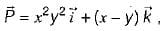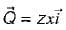φ = xy2z3 then match List-I with List-II and select the correct answer using the codes given below the lists: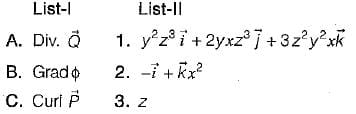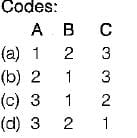Solution:

Here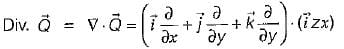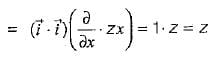Also,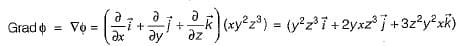And,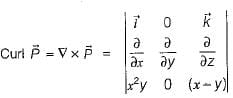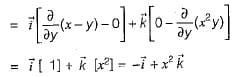QUESTION: 2

### The unit vector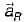which points from z = h on the z-axis towards (r, φ, 0) in cylindrical co-ordinates as shown below is given by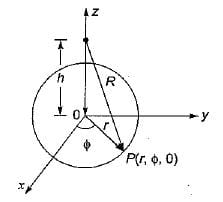Solution:

Let the unit vector be given by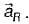Now,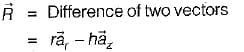∴ Unit vector,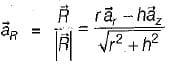QUESTION: 3

### If the vector V given below is irrotational, then the values of a, b and c will be respectively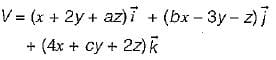Solution:

Since the given vector is irrotational, therefore curl V = 0 or,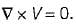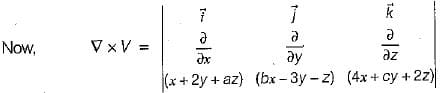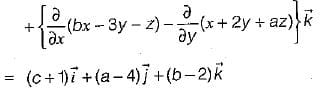Since,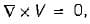therefore, a = 4, b = 2, and c = -1

QUESTION: 4

Match List-I (Vector Identities) with List-ll. (Equivalent expression) and select the correct answer using the codes given below the lists: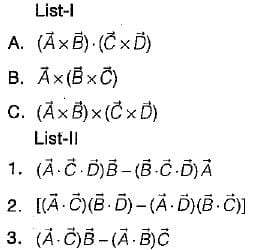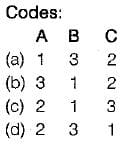Solution:
•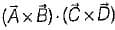is called “ product of four vectors ”.
•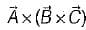is called “vector triple product”.
•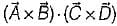is called "vector product of four vectors ”.
QUESTION: 5

The vector differential operator,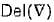in spherical co-ordinate system is given by

Solution:
QUESTION: 6

Assertion (A): Divergence of a vector function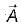at each point gives the rate per unit volume at which the physical entity is issuing from that point.

Reason (R): If some physical entity is generated or absorbed within a certain region of the field, then that region is known as source or sink respectively and if there are no sources or sinks in the field, the net outflow of the incompressible physical entity over any part of the region is zero. However, the net outflow is said to be positive, if the total strength of the sources are greater than the total strength of sink and vice-versa.

Solution:

Both assertion and reason are true and reason is the correct explanation of assertion. Reason is the physical interpretation of divergence.

QUESTION: 7

Which of the following identity is not true?

Solution:
•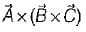is called “vector triple product" which is a correct expression.
•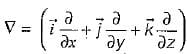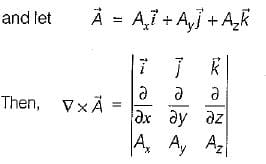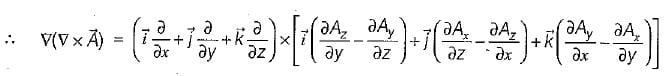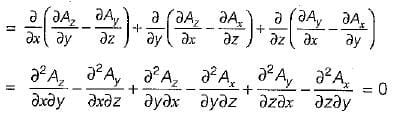•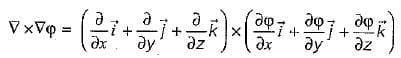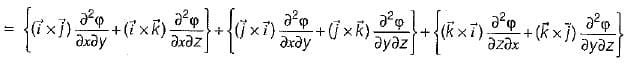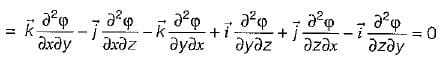QUESTION: 8

The vector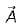directed from (2, - 4,1) to (0, -2,0) in Cartesian coordinates is given by

Solution:

The vector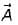is given as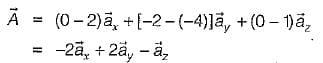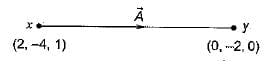QUESTION: 9

What is the value of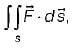where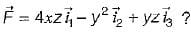Here, s is the surface bounded by x = 0, x = 1, y = o, y = 1, z = 0, z =1 and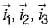are unit vectors along x, yand z axes respectively.

Solution:

By divergence theorem,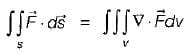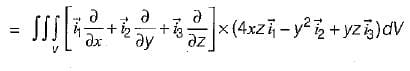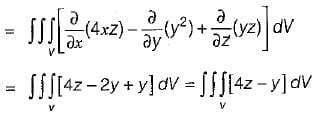Since, the surface s is bounded by x = 0, 1; y = 0, 1 and z = 0, 1 so, putting the limits, we have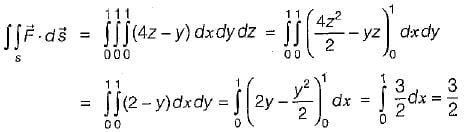QUESTION: 10

The vector field given by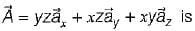Solution: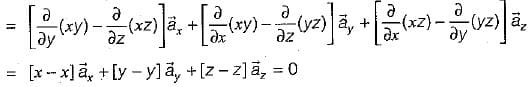The vector field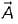will be irrotational,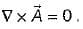Now,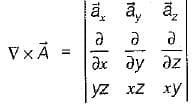Hence,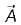is irrotationai.
The vector field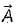will be solenoidal,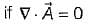Here,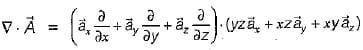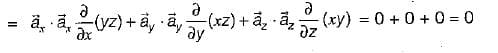Hence,is solenoidal.Use Code STAYHOME200 and get INR 200 additional OFF Use Coupon Code